## “Time Series modeling is a powerful technique that acts as a gateway to understanding and forecasting trends and patterns. But even a time series model has different facets. Most of the examples we see on the web deal with univariate time series. Unfortunately, real-world use cases don’t work like that. There are multiple variables at play, and handling all of them at the same time is where a data scientist will earn his worth. In this article, we will understand what a multivariate time series is, how to deal with it and how we can develop different methodologies to process and perform time-series analysis.”

Forecasting is basically making predictions about the future which plays a key role in the decision-making process of any company that wants to maintain a successful business. This is due to the fact that success tomorrow is determined by the decisions made today, which are based on forecasts. Hence good forecasts are crucial, for example, for predicting sales to better plan inventory, forecasting economic activity to inform business development decisions, or even predicting the movement of people across an organization to improve personnel planning.

Forecasting any future entity can consist of entities having multiple variables or features in its own. For example, a sequence of entities can be represented as : X1, X2, X3, … ,Xn for n time-steps. Here each Xi can consist of multiple features in itself, i.e each Xi may be represented as Xi = (x1,x2,…..xr).

Univariate vs Multivariate Time-Series:

As discussed above, the entities which are being forecasted using different models may have ‘r’ number of features, and thus for predicting an entity all its features are required to be predicted. Time-series forecasting models may have different performance in predicting only one feature in each time-step vs predicting ‘r’ features in each time-step as the errors may start to accumulate as we increase the number of features.

The parameter ‘r’ brings the distinction between univariate and multivariate time-series, in case of univariate time-series r = 1 while for multivariate time-series r >= 2. A univariate time series, as the name suggests, is a series with a single time-dependent variable. For example, have a look at the sample dataset below that consists of the temperature values (each hour), for the past 2 years. Here, temperature is the dependent variable (dependent on Time).If we are asked to predict the temperature for the next few days, we will look at the past values and try to gauge and extract a pattern. We would notice that the temperature is lower in the morning and at night, while peaking in the afternoon. Also if you have data for the past few years, you would observe that it is colder during the months of November to January, while being comparatively hotter in April to June. Such observations will help us in predicting future values. Did you notice that we used only one variable (the temperature of the past 2 years,)? Therefore, this is called Univariate Time Series Analysis/Forecasting.

A Multivariate time series has more than one time-dependent variable. Each variable depends not only on its past values but also has some dependency on other variables. This dependency is used for forecasting future values. Now suppose our dataset includes perspiration percent, dew point, wind speed, cloud cover percentage, etc. along with the temperature value for the past two years. In this case, there are multiple variables to be considered to optimally predict temperature. A series like this would fall under the category of multivariate time series. Below is an illustration of this:Managing Multivariate Time-Series using VAR:

Vector Auto Regression (VAR) is one of the commonly used methods for handling Multivariate Time-Series. In a VAR model, each variable is a linear function of the past values of itself and the past values of all the other variables. To understand it in a better way let us use a visual example:

We have two variables, y1 and y2. We need to forecast the value of these two variables at time t, from the given data for past n values. For simplicity, let us consider the lag value to be 1.For calculating y1(t), we will use the past value of y1 and y2. Similarly, to calculate y2(t), past values of both y1 and y2 will be used. Below is a simple mathematical way of representing this relation: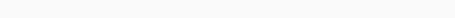– (1)– (2)

Here, a1 and a2 are constant terms, w11, w12, w21, and w22 are the coefficients and e1 and e2 are the error terms. These equations are similar to the equation of an AR(Auto-Regression) process. Since the AR process is used for univariate time series data, the future values are linear combinations of their own past values only. Consider the AR(1) process:

y(t) = a + w*y(t-1) +e

In this case, we have only one variable ‘y’, a constant term ‘a’, an error term ‘e’, and a coefficient ‘w’. In order to accommodate the multiple variable terms in each equation for VAR, we will use vectors.  We can write the equations (1) and (2) in the following form :The two variables are y1 and y2, followed by a constant, a coefficient metric, lag value, and an error metric. This is the vector equation for a VAR(1) process. For a VAR(2) process, another vector term for time ‘t-2’ will be added to the equation to generalize for p lags:The above equation represents a VAR(p) process with variables y1, y2 …yk. The same can be written as: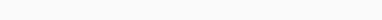The term εt in the equation represents multivariate vector white noise. For a multivariate time series, εt should be a continuous random vector that satisfies the following conditions:

• The expected value of the error vector is 0, i.e E(εt) = 0

• The expected value of εt and εt‘ is the standard deviation of the series, i.e E(εt1,εt2‘) = σ12

What is the need of VAR?

If we recall the temperature forecasting example, an argument can be made for it to be treated as a multiple univariate series. We can solve it using simple univariate forecasting methods like AR. Since the aim is to predict the temperature, we can simply remove the other variables (except temperature) and fit a model on the remaining univariate series. Another simple idea is to forecast values for each series individually using the techniques we already know. This would make the work extremely straightforward! Then why should you learn another forecasting technique?

From the above equations (1) and (2), it is clear that each variable is using the past values of every variable to make the predictions. Unlike AR, VAR is able to understand and use the relationship between several variables. This is useful for describing the dynamic behavior of the data and also provides better forecasting results.

Stationarity of Multivariate Time-Series:

We know from studying the univariate concept that a stationary time series will more often than not give us a better set of predictions. For a given univariate time series:

y(t) = c*y(t-1) + ε t

The series is said to be stationary if the value of |c| < 1. Now, recall the equation of our VAR process: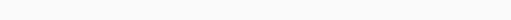Here I is the Identity Matrix.

Representing the equation in terms of Lag Operators, we get :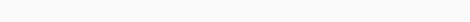Taking all the y(t) terms on the left-hand side: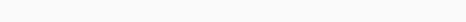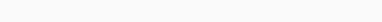The coefficient of y(t) is called the lag polynomial. Let us represent this as Φ(L), we have: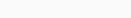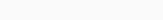For a series to be stationary, the eigenvalues of |Φ(L)-1| should be less than 1 in modulus. For better understanding about stationarity one may refer to the following link.